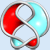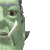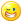## Welcome to the Second Life Forums Archive

These forums are CLOSED. Please visit the new forums HERE

# Parametric Surface Rezzer

Seifert Surface
MathematicianJoin date: 14 Jun 2005
Posts: 912
11-21-2006 01:00
This is mirrored in the lslwiki.

The following is a set of scripts for rezzing surfaces from arbitrary parametric equations.

Pictures: 1, 2, 3.
CODE
`//Parametric Surface Rezzer//by Seifert Surface//Nov 2006//////PARAMETERS////////Example surface, WARNING: rezzes 200 primsfloat scale = 1.0;     //scales the surface in all directions. //If this is 2.0 the surface will be twice as large as if it is 1.0float depth = 0.01;  //thickness of triangles rezzedfloat min_u = -4.0;float max_u = 4.0;integer steps_u = 10;float min_v = -4.0;float max_v = 4.0;integer steps_v = 10;vector f(float u, float v)   //the parametric function{    return <u, v, 2.0 * llSin(u) * llSin(v)>;}//////END OF PARAMETERS////////Description://This collection of scripts rezzes a surface defined by a parametric //function. The surface is made out of triangles, one prim per triangle. //There will be at most 2*steps_u*steps_v triangles in the final surface //(it is possible to have fewer triangles as the script does not rez //degenerate triangles). To change the surface rezzed, edit the //PARAMETERS section above.//Do whatever you want with this script. I'll be annoyed if someone //tries to sell it to newbies, but I think the chances of that are slim!//Instructions: //There are three scripts. The scripts "surface_rezzer" (this script), //and "triangle_detailer" go in the prim that will do the building. //Another script, "triangle_rezzee" goes inside another prim, which //must be called "triangle".  The prim "triangle" goes in the inventory //of the builder prim. //To build the surface, touch the builder prim. The surface will be built//centered on the location of the builder prim when touched. Be careful //when rezzing surfaces near the ground, as the builder can get stuck//trying to move to a position to rez something when that position is //underground.//Once the surface is rezzed, the following commands can be shouted //on channel 347://"die" - Deletes all rezzed triangles (in range of your shout).//"clear" - removes scripts from all rezzed triangles (in range of your //shout).//If you prefer, you can have the triangles remove their scripts as soon //as they are in position, there is a commented-out line in the triangle //rezzee script which can be uncommented to do this.//Have fun!// Seifert Surface// 2G!tGLf 2nLt9cGinteger comm_channel = -61347732;integer rez_counter;vector origin;float delta = 0.01;rez_triangle(vector a, vector b, vector c){	a = origin + scale * a;	b = origin + scale * b;	c = origin + scale * c;	//check for 2 vertices in the same place	if(llVecDist(a,b) < delta || llVecDist(b,c) < delta || llVecDist(c,a) < delta)	{  //if so, don't try to rez the triangle		return;	}	float cosA = (b - a) * (c - a);	float cosB = (c - b) * (a - b);	float cosC = (a - c) * (b - c);  //signs -ve means obtuse angle	if(cosA < 0.0)  //so the angle at a is obtuse, meaning the opposite edge must be the base of the prim	{		triangle(a, b, c, TRUE);	}	else if(cosB < 0.0)	{		triangle(b, c, a, TRUE);	}	else if(cosC < 0.0)	{		triangle(c, a, b, TRUE);	}	else //all acute angles... so we can choose which way to base the prim, so as to minimise the	{		//error introduced by the shear value having a resolution of only 0.01		float error1 = triangle(a,b,c,FALSE);		float error2 = triangle(b,c,a,FALSE);		float error3 = triangle(c,a,b,FALSE);		if(error1 < error2)		{			if(error1 < error3)			{				triangle(a,b,c,TRUE);			}			else 			{				triangle(c,a,b,TRUE);			}		}		else		{			if(error2 < error3)			{				triangle(b,c,a,TRUE);			}			else			{				triangle(c,a,b,TRUE);			}		}	}}         float triangle(vector a, vector b, vector c, integer rez){		float width = llVecDist(b, c);	vector left = llVecNorm(b - c);	vector fwd = llVecNorm(left % (a - c));	vector up = fwd % left;	float height = (a - c) * up;	float y_shear = 0.5 - ((b-a) * left) / width;		if(rez)	{		vector  center = 0.5 * ( (b+c) + (height * up)  );		vector scale = <depth, width, height>;		rotation rot = llAxes2Rot(fwd, left, up);		llMessageLinked(LINK_THIS, comm_channel + rez_counter, (string)scale + "|" + (string)y_shear, NULL_KEY);				while(llVecDist(llGetPos(), center) > 9.5)		{			llSetPos(center);		}		llRezObject("triangle", center, ZERO_VECTOR, rot, comm_channel + rez_counter);		rez_counter += 1;		return -1.0;	}	else	{		y_shear = (float)llRound(y_shear * 100.0) / 100.0;		return llVecDist(a, 0.5 * (b + c) + height * up + y_shear * width * left); 		//error between where the vertex of the triangle should be and would be...	}}rez_surface(){	integer i;	integer j;	float scale_u = (max_u - min_u) / (float)steps_u; 	float scale_v =  (max_v - min_v) / (float)steps_v; 	for(i=0; i<steps_u; i+=1)	{		for(j=0; j<steps_v; j+=1)		{			vector A = f(min_u + scale_u * i, min_v + scale_v * j);			vector B =  f(min_u + scale_u * (i + 1), min_v + scale_v * j);			vector C =  f(min_u + scale_u * i , min_v + scale_v * (j + 1));			vector D =  f(min_u + scale_u * (i + 1) , min_v + scale_v * (j + 1));			rez_triangle(A, B, C);			rez_triangle(B, D, C);			//llSleep(0.2); //in some very laggy situations this may be necessary to add, shouldn't be necessary			//only use if the triangle_detailer gets backed up on its list.		}	}}default{    touch_start(integer num)    {        if(llDetectedKey(0) == llGetOwner())        {            origin = llGetPos();			rez_surface();			while(llVecDist(llGetPos(), origin) > 9.5) 			{//go back to the start				llSetPos(origin);			}			llSetPos(origin);			llOwnerSay("Done!");        }    }}`
CODE
`//Triangle Detailer//by Seifert Surface//Nov 2006integer comm_channel2 = 61347732;list rezzees;list tri_data;default{    state_entry()    {        llListen(comm_channel2, "", NULL_KEY, "");    }    link_message(integer sender, integer num, string str, key id)    {		rezzees += [num];		tri_data += [str];		if(llGetListLength(rezzees) > 10)		{			llSay(DEBUG_CHANNEL, "Detailer list getting long...");		}    }    listen(integer chan, string name, key id, string msg)    {        //llOwnerSay((string)llGetFreeMemory() + " " + (string)llGetListLength(rezzees));  //in case you want to see if the detailer is getting overloaded        integer who = llListFindList(rezzees, [(integer)msg]);        if(who != -1)        {            llShout((integer)msg, llList2String(tri_data, who));            rezzees = llDeleteSubList(rezzees, who, who);            tri_data = llDeleteSubList(tri_data, who, who);        }    }}`
CODE
`//Triangle Rezzee//by Seifert Surface//Nov 2006integer comm_channel = -61347732;integer comm_channel2 = 61347732;integer me;integer listen_control;integer times_to_ask = 10;default{    state_entry()    {        llListen(347, "", "", "");    }    on_rez(integer param)    {        if(param != 0)        {            me = param;            llSetObjectName((string)(me - comm_channel));            listen_control = llListen(me, "", "", "");            llShout(comm_channel2, (string)me);            llSetTimerEvent(5.0);        }    }    listen(integer chan, string name, key id, string msg)    {        if(msg == "die")        {            llDie();        }        else if(msg == "clear")        {            llRemoveInventory(llGetScriptName());           }        else if(chan == me)        {            list data = llParseStringKeepNulls(msg, ["|"], []);            vector scale = (vector)llList2String(data, 0);            if(scale.x < 0.01)            {                scale.x = 0.01;            }            if(scale.y < 0.01)            {                scale.y = 0.01;            }            if(scale.z < 0.01)            {                scale.z = 0.01;            }            float y_shear = (float)llList2String(data, 1);            llSetPrimitiveParams([PRIM_TYPE, PRIM_TYPE_BOX, 0, <0.0, 1.0, 0.0>, 0.0, <0.0, 0.0, 0.0>, <1.0, 0.0, 0.0>, <0.0, y_shear, 0.0>, PRIM_SIZE, scale]);              //llRemoveInventory(llGetScriptName());   //if you want to remove scripts as soon as possible, uncomment this.			llListenRemove(listen_control);            llSetTimerEvent(0.0);        }    }    timer()    {        llShout(comm_channel2, (string)me);        times_to_ask -= 1;        if(times_to_ask <= 0)        {            llSetTimerEvent(0.0);            llSay(DEBUG_CHANNEL, "no reply after 10 tries");           }    }}`
Here are some example parametric equations to plug in. The number of prims used can be reduced by lowering the steps_u and steps_v variables, though the surfaces will not look as good. It is also a good idea to lower the scale number as well, to avoid prims trying (and failing) to scale to above 10m.
CODE
`//Sphere, with a slight twist //224 primsfloat depth = 0.01;float scale = 3.0;float min_u = 0.0;float max_u = TWO_PI;integer steps_u = 16;float min_v = 0.0;float max_v = PI;integer steps_v = 8;vector f(float u, float v){    return <llSin(u + 0.5 * v) * llSin(v), llCos(u + 0.5 * v) * llSin(v), llCos(v)>;}`
CODE
`//Klein Bottle//800 prims, large (rez in a sandbox)float depth = 0.01;float scale = 5.0;float min_u = 0.0; float max_u = TWO_PI; integer steps_u = 20;float min_v = 0.0;float max_v = TWO_PI;integer steps_v = 20;float a = 2.0;vector f(float u, float v){    float temp = (a + llCos(u * 0.5) * llSin(v) - llSin(u*0.5)*llSin(2*v));    return < temp * llCos(u), temp * llSin(u), llSin(0.5*u)*llSin(v) + llCos(0.5*u) * llSin(2*v)>;}`
CODE
`//Dini's Surface//800 prims, large (rez in a sandbox)float depth = 0.01;float scale = 10.0;float min_u = -5.0;float max_u = 5.0;integer steps_u = 20;float min_v = -3.14159;float max_v = PI;integer steps_v = 20;vector f(float u, float v){    float psi = 1.2; //this can be from 0 to PI    float sinpsi = llSin(psi);    float cospsi = llCos(psi);    float g = (u - cospsi * v) / sinpsi;    float s = llPow(2.7182818, g);    float r = (2 * sinpsi) / (s + 1 / s);    float t = r * (s - 1 / s) * 0.5;        return <u - t, r * llCos(v), r * llSin(v)>;}`
CODE
`//Boy's surface//1250 prims, largefloat depth = 0.01;float scale = 25.0;float min_u = 0.0;float max_u = PI;integer steps_u = 25;float min_v = 0.0;float max_v = PI;integer steps_v = 25;float E = 2.718281828;float Exp(float x){    return llPow(E, x);}float Cosh(float x){    return 0.5 * (Exp(x) + Exp(-x));}float Sinh(float x){    return 0.5 * (Exp(x) - Exp(-x));}vector f(float u, float v){    float x = llCos(u) * llSin(v);    float y = llSin(u) * llSin(v);    float z = llCos(v);    float ef = 0.5 * ( (2 * x*x - y*y - z*z) + 2*y*z*(y*y - z*z) + z*x*(x*x - z*z) + x*y*(y*y - x*x) );    float g = llSqrt(3.0) * 0.5 * ( (y*y - z*z) + z*x*(z*z - x*x) + x*y*(y*y - x*x) );    float h = (x+y+z) * ( llPow(x+y+z, 3.0) + 4 * (y - x)*(z - y)*(x - z)  );    return <ef,g,h * 0.125>;}`
_____________________
-Seifert Surface
2G!tGLf 2nLt9cG
Nada Epoch
The LibrarianJoin date: 4 Nov 2002
Posts: 1,423
Discussion Thread
11-23-2006 09:54
/54/30/150639/1.html
_____________________
i've got nothing.